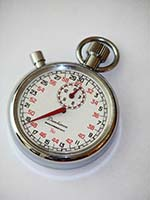Goddard Space Flight CenterLesson Plans: Time that Star!Time that Star!

Estimated class time: 2-3 class periods

Lesson plan by Kara C. Granger of Maria Carillo High School in Santa Rosa, CA

Summary

This lesson examines the idea of periodic behavior and how it is determined from a set of data. The students will examine this concept through a hands-on lab that involves a simple, student-made pendulum which is followed up with critical thinking and performance assessment style questions. Students will also analyze data received from high-energy satellites of various binary star systems' orbital periods or stellar rotation periods.

Objectives

1. Students will recognize a periodicity within a set of data.
2. Students will learn how to complete and apply the method of Folding to finding the period of the periodicity.
3. Students will apply the method of Folding to determine the periodicity of pendulums, sample data, and orbits of binary star systems.
4. Students will learn an appreciation for basic astronomy and astrophysics through the exposure of such topics embedded in this lesson.

National Education Standards

National Science Education Standards

This lesson plan addresses the following National Science Education Standards:

NS.5-8.1 Science as Inquiry
As a result of activities in grades 5-8, all students should develop:

1. Abilities necessary to do scientific inquiry

NS.5-8.2 Physical Science
As a result of their activities in grades 5-8, all students should develop an understanding:

1. Motions and forces
2. Transfer of energy

NS.9-12.1 Science as Inquiry
As a result of activities in grades 5-8, all students should develop:

1. Abilities necessary to do scientific inquiry

NS.9-12.2 Physical Science
As a result of their activities in grades 9-12, all students should develop an understanding of:

1. Motions and forces
2. Conservation of energy and increase in disorder

NS.9-12.4 Earth and Space Science
As a result of activities in grades 5-8, all students should develop an understanding of:

1. Origin and evolution of the universe

National Council of Teachers of Mathematics

This lesson plan addresses the following educational standards from the National Council of Teachers of Mathemantics:

• Process Standards
• Problem Solving
Instructional programs from prekindergarten through grade 12 should enable all students to:
1. Build new mathematical knowledge through problem solving
2. Solve problems that arise in mathematics and in other contexts
• Connections
Instructional programs from prekindergarten through grade 12 should enable all students to:
1. Recognize and apply mathematics in contexts outside of mathematics
• Algebra
• Understand patterns, relations, and functions
In grades 6-8 all students should:
1. represent, analyze, and generalize a variety of patterns with tables, graphs, words, and, when possible, symbolic rules
In grades 9-12 all students should:
1. generalize patterns using explicitly defined and recursively defined functions
2. interpret representations of functions of two variables
• Represent and analyze mathematical situations and structures using algebraic symbols
In grades 6-8 all students should:
1. use symbolic algebra to represent situations and to solve problems, especially those that involve linear relationships
In grades 9-12 all students should:
1. use symbolic algebra to represent and explain mathematical relationships
• Use mathematical models to represent and understand quantitative relationships
In grades 6-8 all students should:
1. model and solve contextualized problems using various representations, such as graphs, tables, and equations
• Data Analysis
• Select and use appropriate statistical methods to analyze data
In grades 6-8 all students should:
1. b. discuss and understand the correspondence between data sets and their graphical representations, especially histograms, stem-and-leaf plots, box plots, and scatterplots
• Formulate questions that can be addressed with data and collect, organize and display relevant date to answer them
In grades 9-12 all students should:
1. understand histograms, parallel box plots, and scatterplots and use them to display data

Prerequisites

• Students should know how to graph/plot data and determine the mean from a set of data.
• Students should have been exposed to a variety of astronomy and astrophysical concepts, such as those discussed in the Science section of the Imagine the Universe! Web site. Information in the Introduction section of this lesson's Day 2 can also be used.

Procedure

This lesson has been developed as a multi-day plan. Each lesson day has been designed to take approximately an hour to complete. The lesson is broken down as follows:

• Day 1: Students complete a hands-on lab involving a pendulum and recognition of periodic behavior
• Day 2: Students apply the method of Folding to determine a period in sample data and from actual satellite data
• Enrichment: Day 2 includes an optional enrichment where students learn and apply the chi-square test to determine the period in a set of data

References

• Kaufmann, William J. III, Universe, Freeman and Company, 1994, pgs. 336-435
• Kerrod, Robin, Encyclopedia of Science: The Heavens Stars, Galaxies, and the Solar System, Macmillan Publishing Company, 1991
• Kondo, Herbert, The New Book of Popular Science Vol. 1, Grolier Incorporated, 1982, pgs. 174-205
• Sanford, Peter W. and Salton, Jane and Laskarides, Paul, Galactic X-ray Sources, John Wiley and Sons, 1982 pgs. 161-162
• Seward, Frederick D. and Charles, Philip A., Exploring the X-ray Universe, Cambridge University Press, 1995

Data used in this lesson were retrieved from the HEASARC Data Archive.A service of the High Energy Astrophysics Science Archive Research Center (HEASARC), Dr. Alan Smale (Director), within the Astrophysics Science Division (ASD) at NASA/GSFC